Uh Oh! It seems you’re using an Ad blocker!

We always struggled to serve you with the best online calculations, thus, there's a humble request to either disable the AD blocker or go with premium plans to use the AD-Free version for calculators.

Or# Surface Area Calculator

Shape:

Base Shape:

Let pi πHeight h:

Table of Content

 1 What is priceeight Class? 2 priceeight Class Chart: 3 How to Calculate priceeight Density (Step by Step): 4 Factors that Determine priceeight Classification: 5 What is the purpose of priceeight Class? 6 Are mentioned priceeight Classes verified by the officials? 7 Are priceeight Classes of UPS and FedEx same?

Get the Widget!

Add this calculator to your site and lets users to perform easy calculations.

Feedback

How easy was it to use our calculator? Did you face any problem, tell us!

Our Surface Area Calculator will find the surface area of three-dimensional solids like Capsules, Spheres, Cylinders, Cone, Prism, Pyramids, Hemisphere, Ellipsoid, etc.

## How does This Surface Area Calculator Work?

We do need to follow the guidelines as under to use the surface area solver.

Input:

• Choose the Geometrical shape from the drop-down menu.
• Insert the relevant data for each shape.
• Hit the calculate button to find the surface area.

Output:

• The surface area of the geometric shape.

## What is Surface Area and How to Find it?

The measure of the total area covered or occupied by an object is known as the surface area of a solid geometrical shape.

A total surface area calculator or different formulas are used for finding the surface area of various solids. The surface area formula has two distinct parts: the base area and the lateral area.

### Base Area:

Base area refers to the area of the base of a solid. It can be different for various solids, as it can be rectangular, square, circular, triangular, etc.

### Lateral Surface Area:

The lateral surface is the surface area of all the sides of the solids excluding the base and the top. You can measure the lateral surface area using the lateral surface area calculator.

## Surface Area Formulas for Various Geometrical Shapes

Below are the surface area formulas for various geometric shapes, have a look at them.

### The Surface Area of Sphere:

For finding the surface area of the sphere, you need to find the sphere’s radius or its diameter. You can also use a surface area finder to get the radius or diameter.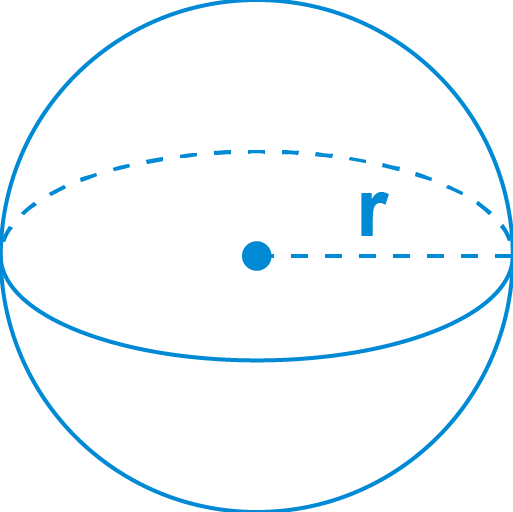The formula for the surface area of a sphere is:

• S.A = 4 * π * r²
• Or
• S.A =  π * d²

Where:

d is the diameter

### The Surface Area of Cone:

The surface area of a cone can be measured by summing the base surface area and the lateral surface area. You can also use the surface area of pyramids and cones calculator for more accurate results.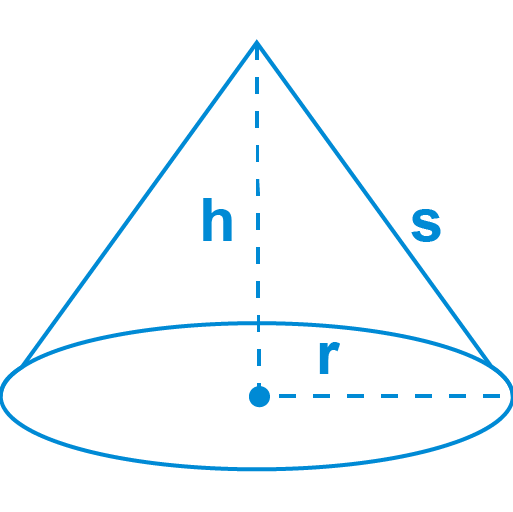base SA = πr2

lateral SA = πr√r2 + h2

total SA = πr(r + √r2 + h2)

Where

r is the radius and h is the height

### The Surface Area of Cube:

The Surface area of a cube can be measured by finding the area of a side and multiplying it by six.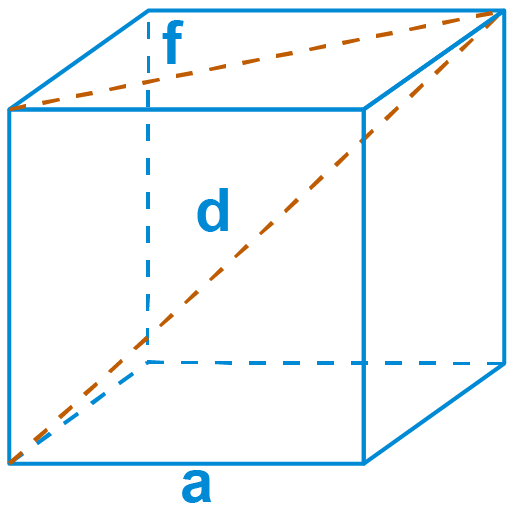The formula for the Surface area of a cube:

SA = 6a2

Where:

a is the edge length

This formula is also used by the surface area of prism calculator.

### The Surface Area of Cylinder:

The surface area of the cylinder can be measured by summing the base area and the lateral surface area of the cylinder. You can also use the surface area of prism and cylinders calculator to do this task efficiently.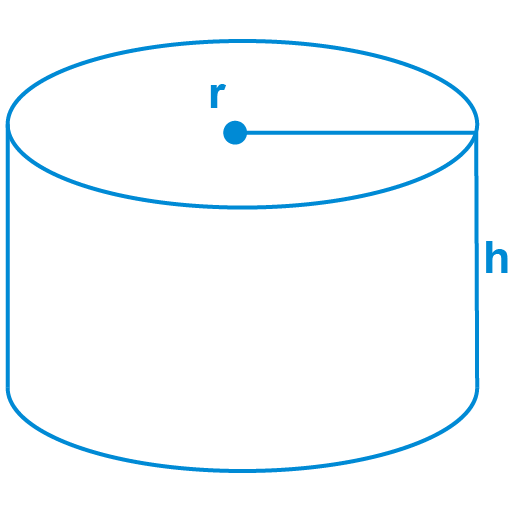The formula for the Surface area of a cylinder:

base SA = 2πr2

lateral SA = 2πrh

total SA = 2πr(r + h)

where

r is the radius and h is the height

### The Surface Area of Rectangular Prism:

To measure the surface area of a Rectangular Prism, consider the area of three rectangular sides. You can find the area of the Rectangular Prism using the surface area of a rectangle calculator.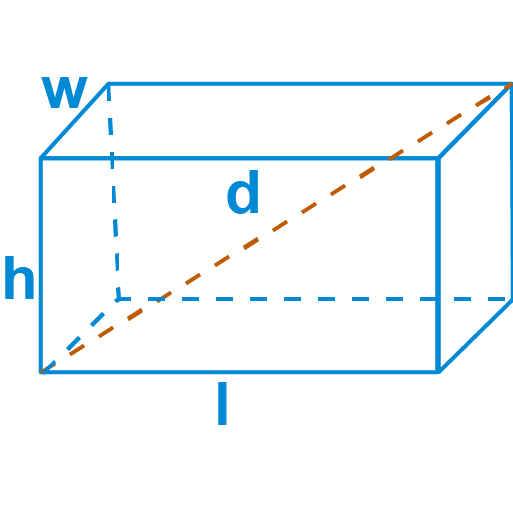SA of  = 2 * (A1 + A2 + A3)

where:

• A1 = l * w
• A2 = w * h
• A3 = l * h

The formula for the Surface area of a cylinder:

A = 2 * (l * w + w * h + l * h)

### The Surface Area of Capsule:

A capsule surface can be calculated by merging the surface area of the sphere and the lateral surface area of the cylinder.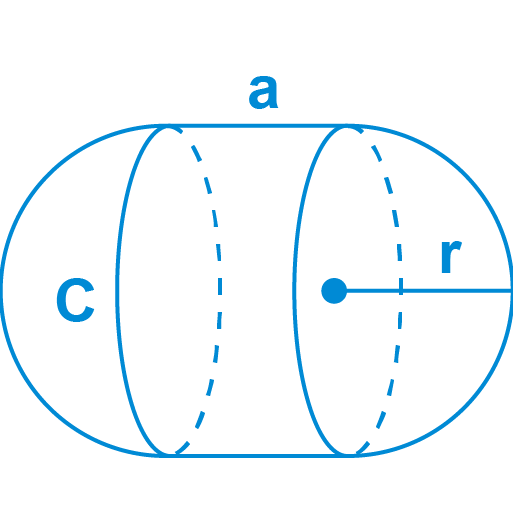The formula for the Surface area of a capsule:

SA = 4πr2 + 2πrh

Where:

h is the height

### The Surface Area of Hemisphere:

The surface area of the hemisphere is the curved surface of the hemisphere plus the base area of the circular portion.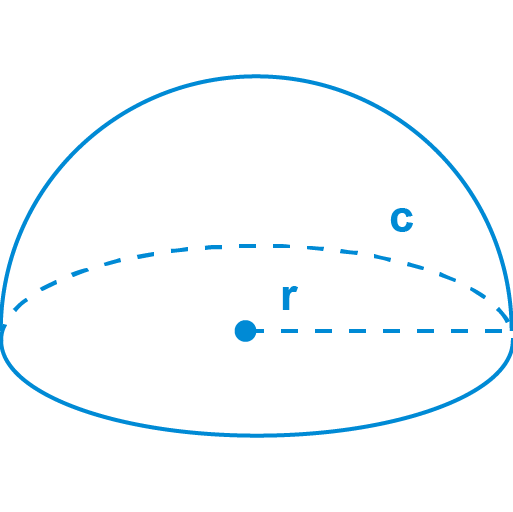The surface area of the Hemisphere = Curved surface area of hemisphere + circular base area

The curved surface area of the hemisphere = 2πr^2

Circular base area = πr^2

Then

Surface area of the Hemisphere = 2πr^2 + πr^2

Thus,

The surface area of a hemisphere = 3πr^2 square units

Where:

“r” is the radius of the hemisphere.

### The Surface Area of Conical Frustum:

The surface area of the conical frustum is the sum of two circular portions and the lateral face.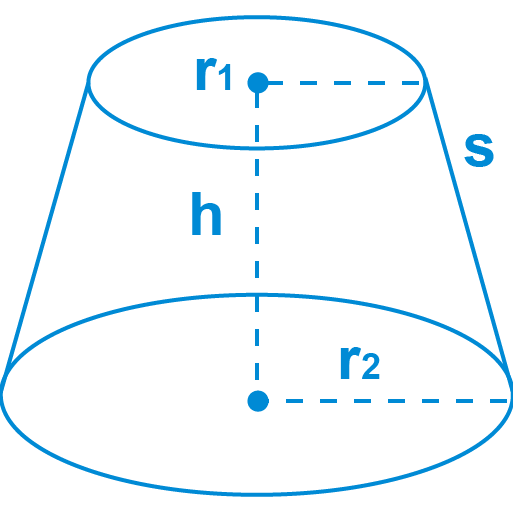The formula for the Surface area Conical Frustum:

The area of Conical Frustum  = Circle Top + Circular Bottom

Circle top = π  r2

Circular bottom = π  R2

Then

Area of Circular end SA = π(R2 + r2)

lateral SA = π(R+r)√(R-r)2 + h2

total SA = π(R2 + r2) + π(R+r)√(R-r)2 + h2

Where:

R and r are the radii of the ends

h is the height

### The Surface Area of the Spherical Cap:

There are two portions of the Surface area of the Spherical Cap, the spherical cap and the base area. The Spherical Cap includes the height “h” and the area of the circle, and then multiply it by the height.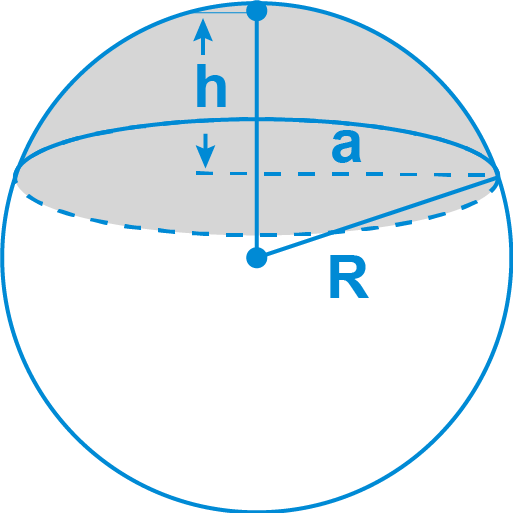The formula for the Surface area Spherical cap:

Spherical cap SA = 2πRh

Base SA = πr2

SA of Spherical Cap= 2πRh + πr2

### The Surface Area of Triangular Prism:

The surface area of a Triangular prism can be determined by the perimeter of the face of the Triangular Prism. Now multiply the perimeter by the length and add the base area to it.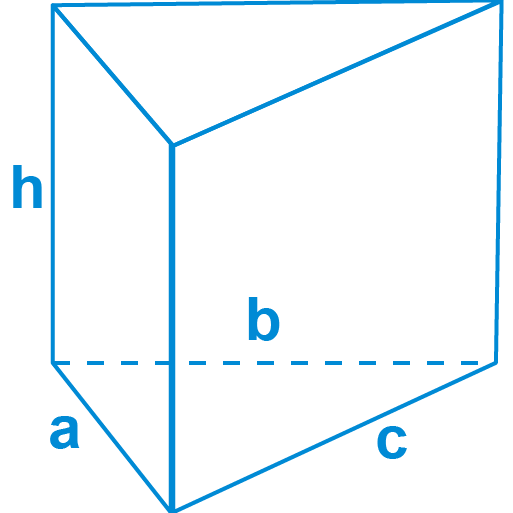The formula for the Surface area Triangular Prism:

The SA of Triangular prism = (perimeter * length)+ (2 * base area)

### The Surface Area of the Pyramid:

The various pyramids can be of different shapes due to their base, the pyramids can be subdivided into various types on the basis of their base shape.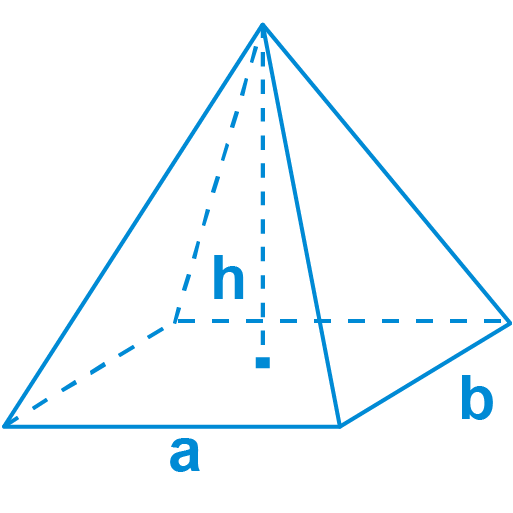A = A(base) + A(lateral) = A(base) + 4 * A(lateral face)

In the case of the square base

Lateral Surface Area = a√(a2 + 4h2)

Base Surface Area = a2

Total Surface Area= L + B = a2 + a√(a2 + 4h2))

S.A Pyramid = A(base) + A(lateral) = A(base) + 4 * A(lateral face)
S.A Pyramid  =  a(a + √(a2 + 4h2))

It can be a little difficult when you are dealing with the various geometrical-shaped pyramids. The surface area of the pyramid can be measured by the surface area of a pyramid calculator

### The Surface Area of Ellipsoid:

The Ellipsoid Surface area is not simple, you need to learn about the ellipse shape and the vertices.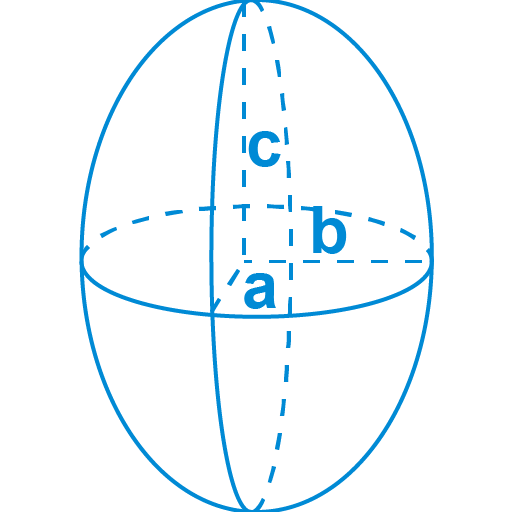The formula for the Surface area Ellipsoid:

SA ≈ 4π 1.6√(a1.6b1.6 + a1.6c1.6 + b1.6c1.6)/3

where a, b, and c are the axes of the ellipse

## References:

From the source of Wikipedia: Surface area,Definition

From the source of the tutorial.math.lamar.edu: Solid Area, Formula of SA